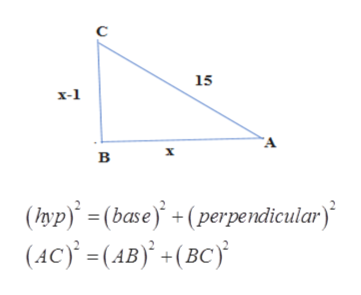# The length of one leg of a right triangle is 1 m shorter than the other. If the hypotenuse is 5m long, what are the lengths of the sides?

Question
4 views

The length of one leg of a right triangle is 1 m shorter than the other. If the hypotenuse is 5m long, what are the lengths of the sides?

check_circle

Step 1

Given, the length of one leg of a right triangle is 1 m shorter than the other and  the hypotenuse is 5m long.

Step 2

Let, the longer leg of the right triangle is = x m

So, the shorter length of the right triangle is = (x-1) m

Step 3

By using the Pythago...help_outlineImage Transcriptioncloseс 15 х-1 A х в (hyp = (base)(perpendicular) (АC) - (АB) - (вс) fullscreen

### Want to see the full answer?

See Solution

#### Want to see this answer and more?

Solutions are written by subject experts who are available 24/7. Questions are typically answered within 1 hour.*

See Solution
*Response times may vary by subject and question.
Tagged in

### Algebra